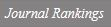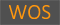On properties of the operator equation TT* = T plus T*

Title
On properties of the operator equation TT* = T plus T*
Authors
An, Il JuKo, Eungil
Ewha Authors
SCOPUS Author ID
고응일Issue Date
2018
Journal Title
FILOMAT
ISSN
0354-5180Citation
FILOMAT vol. 32, no. 6, pp. 2247 - 2256
Keywords
Operator equationsspectrumsingle valued extension property
Publisher
UNIV NIS, FAC SCI MATH
Indexed
SCIE; SCOPUSDocument Type
Article
Abstract
In this paper, we study properties of the operator equation TT* = T + T* which T. T. West observed in . We first investigate the structure of solutions T is an element of B(H) of such equation. Moreover, we prove that if T is a polynomial root of solutions of that operator equation, then the spectral mapping theorem holds for Weyl and essential approximate point spectra of T and f (T) satisfies a-Weyl's theorem for f 2 H(sigma(T)), where H(sigma(T)) is the space of functions analytic in an open neighborhood of sigma(T).
DOI
10.2298/FIL1806247A
Appears in Collections:
자연과학대학 > 수학전공 > Journal papers
Files in This Item:
There are no files associated with this item.
Export
RIS (EndNote)
XLS (Excel)
XML